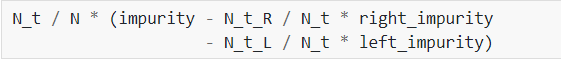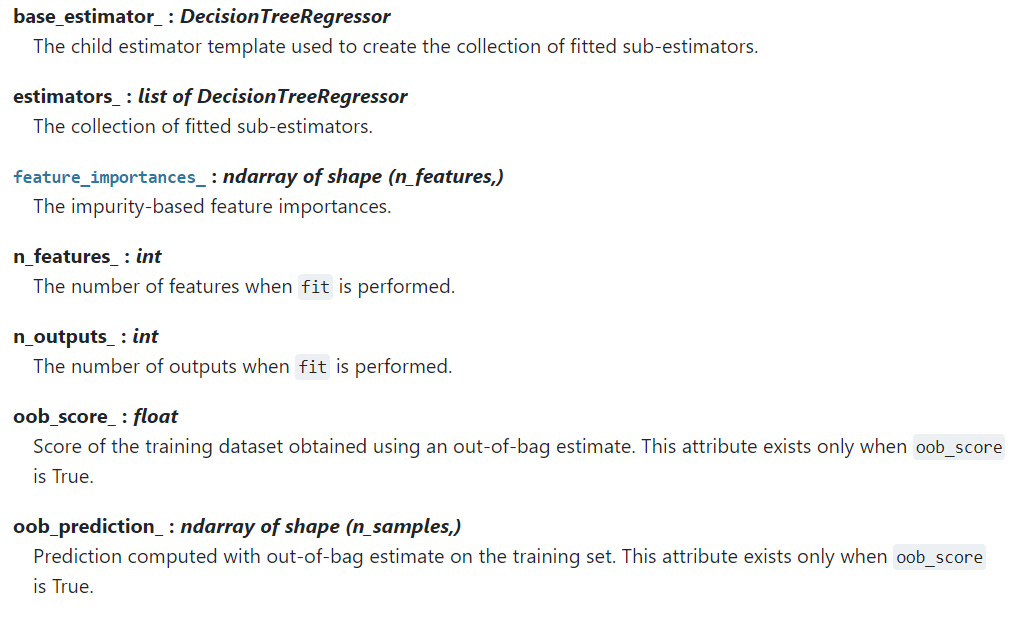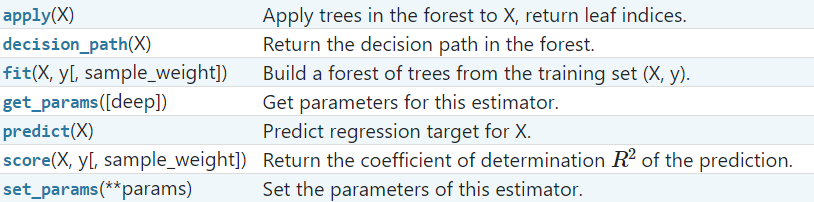# 6.2 API ： RandomForestClassifier与RandomForestRegressor 原创1147-柳同学

### RandomForestClassifier

from sklearn.ensemble import RandomForestClassifier
RandomForestClassifier(n_estimators=100, *, criterion='gini', max_depth=None, min_samples_split=2,
min_samples_leaf=1, min_weight_fraction_leaf=0.0, max_features='auto', max_leaf_nodes=None,
min_impurity_decrease=0.0, min_impurity_split=None, bootstrap=True, oob_score=False, n_jobs=None,
random_state=None, verbose=0, warm_start=False, class_weight=None, ccp_alpha=0.0, max_samples=None)


n_estimators : integer, optional (default=10) 整数，可选择(默认值为10)。

criterion : {“gini”, “entropy”}, default=”gini”

max_depth : int, default=None
（决策）树的最大深度。如果值为None，那么会扩展节点，直到所有的叶子是纯净的(叶节点的所有数据类别都相同)，或者直到所有叶子包含少于min_sample_split的样本。

min_samples_split : int or float, default=2

1. 如果为int，那么考虑min_samples_split作为最小的数字。
2. 如果为float，那么min_samples_split是一个百分比，并且把ceil(min_samples_split*n_samples)是每一个分割最小的样本数量

min_samples_leaf : int or float, default=1

1. 如果为int，那么考虑min_samples_leaf作为最小的数字。
2. 如果为float，那么min_samples_leaf为一个百分比，并把ceil(min_samples_leaf*n_samples)是每一个节点的最小样本数量

min_weight_fraction_leaf : float, default=0.0

max_features : {“auto”, “sqrt”, “log2”}, int or float, default=”auto”

1.如果是int，就要考虑每一次分割处的max_feature特征
2.如果是float，那么max_features就是一个百分比，那么（max_feature*n_features）特征整数值是在每个分割处考虑的。
3.如果是auto，那么max_features=sqrt(n_features)，即n_features的平方根值。
4.如果是log2，那么max_features=log2(n_features)
5.如果是None,那么max_features=n_features

max_leaf_nodes : int, default=None

min_impurity_decrease : float, default=0.0N是样本总的数量，N_t是当前节点处的样本数量，N_t_L是左孩子节点样本的数量,还有N_t_R是右孩子节点的样本数量。
N，N_t，N_t_R和N_t_L全部是指加权总和，如果sample_weight通过的话。

bootstrap : bool, default=True

oob_score : bool, default=False

n_jobs : int, default=None

random_state : int, RandomState instance or None, default=None
random_state是随机数生成器使用的种子; 如果是RandomState实例，random_state就是随机数生成器; 如果为None，则随机数生成器是np.random使用的RandomState实例。

verbose : int, default=0

warm_start : bool, default=False

class_weight : {“balanced”, “balanced_subsample”}, dict or list of dicts, default=None
“balanced_subsample” 或者None,（默认值为None）,与格式{class_label: weight}相关联的类的可选的权值。如果没有给值，所有的类都应该有一个权值。对于多输出问题，一个字典序列可以按照y的列的顺序被提供。

"balanced"模式使用y的值来自动的调整权值，与输入数据中类别频率成反比，如：
n_samples / (n_classes * np.bincount(y))
"balanced_subsample"模式和"balanced"相同，除了权值是基于每棵成长树有放回抽样计算的。

ccp_alpha : non-negative float, default=0.0

max_samples : int or float, default=None

base_estimator_ : DecisionTreeClassifier

estimators_ : list of DecisionTreeClassifier

classes_ : ndarray of shape (n_classes,) or a list of such arrays

n_classes_ : int or list

n_features_ : int

n_outputs_ : int

feature_importances : ndarray of shape (n_features,)

oob_score_ : float

oob_decision_function_ : ndarray of shape (n_samples, n_classes)

apply(X) : 将决策树应用于X，返回叶子索引
decision_path(X) ： 返回森林中的决策路径
fit(X, y[, sample_weight])：从训练集(X, y)中构建一个森林
get_params([deep])：获得这个估计器的参数
predict(X)：预测类x
predict_log_proba(X)：预测X的类对数概率
predict_proba(X)： 预测X的类概率
score(X, y[, sample_weight])：返回给定测试数据和标签的平均精确度
set_params(**params) ： 设置估计器参数

### RandomForestRegressor

from sklearn.ensemble import RandomForestRegressor

RandomForestRegressor(n_estimators=100, *, criterion='mse', max_depth=None, min_samples_split=2,
min_samples_leaf=1, min_weight_fraction_leaf=0.0, max_features='auto', max_leaf_nodes=None,
min_impurity_decrease=0.0, min_impurity_split=None, bootstrap=True, oob_score=False, n_jobs=None,
random_state=None, verbose=0, warm_start=False, ccp_alpha=0.0, max_samples=None)


criterion : {“mse”, “mae”}, default=”mse”

oob_prediction_ : ndarray of shape (n_samples,)### 评论 抢沙发### 觉得文章有用就打赏一下文章作者

#### 支付宝扫一扫打赏#### 微信扫一扫打赏Vieu3.3主题Q Q 登 录